# z1(x) = 2x3 + x ln x, z2(x) = x ln x − x3 are solutions...

z1(x) = 2x3 + x ln x, z2(x) = x ln x − x3 are solutions of a second order, linear nonhomo-geneous equation L[y] = f (x).y1(x) = x−2 is a solution of the corresponding reduced equation L[y] = 0. (a) Give a fundamental set of solutions of the reduced equation L[y] = 0. (b) Give the general solution of the nonhomogeneous equation L[y] = f (x).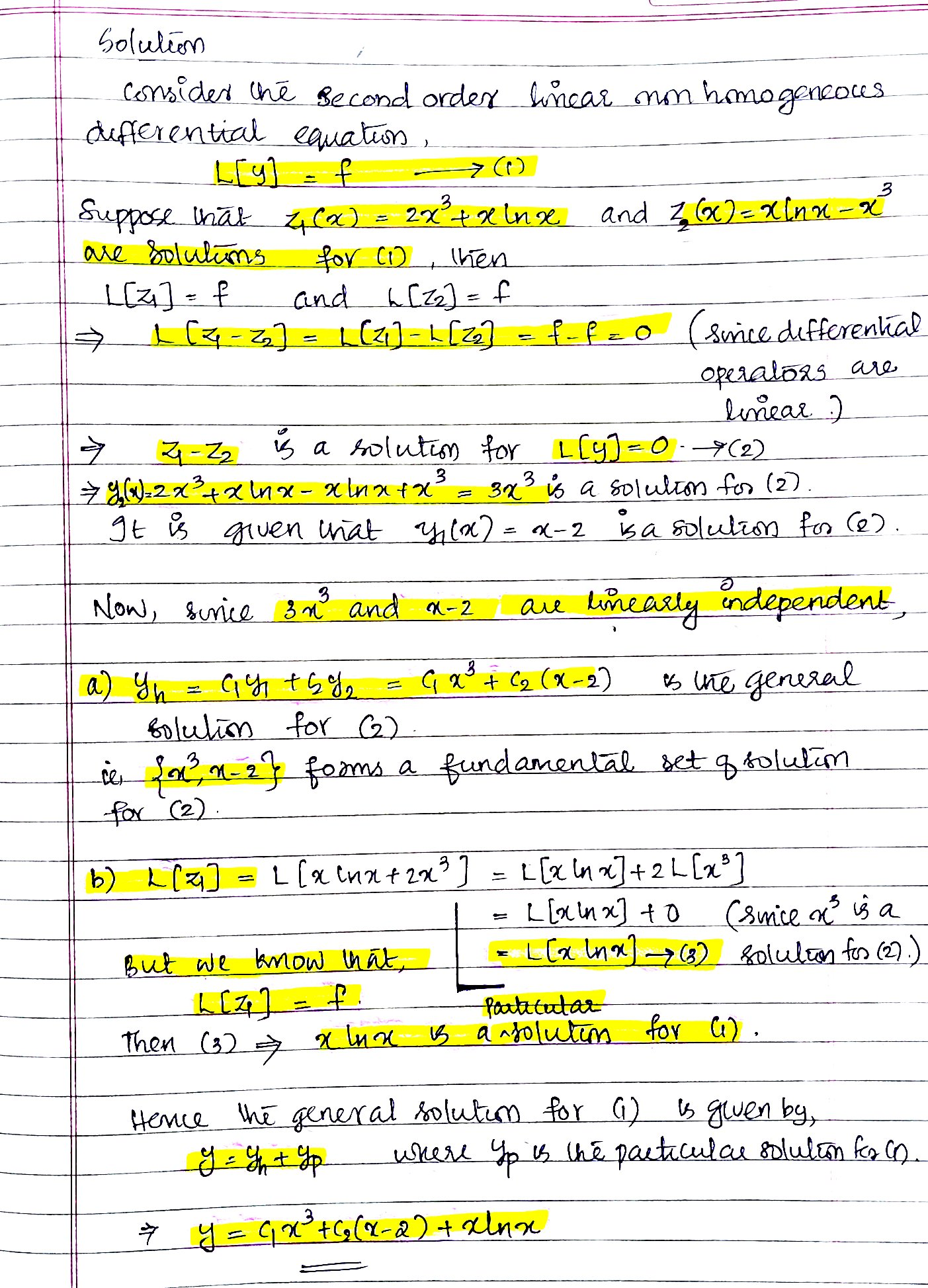C1 should be x^-2, not x^3

#### Earn Coin

Coins can be redeemed for fabulous gifts.

Similar Homework Help Questions
• ### z1(x) = 2x2 + tan x, z2(x) = x2 − 2x + tan x, z3(x) =...

z1(x) = 2x2 + tan x, z2(x) = x2 − 2x + tan x, z3(x) = x2 − 3x + tan x are solutions of a second order, linear nonhomogeneous equation L[y] = f (x). (a) Give a fundamental set of solutions of the corresponding reduced equation L[y] = 0. (b) Give the general solution of the nonhomogeneous equation L[y] = f (x).

• ### {y () = r, yz(2) = r ln r} is a fundamental set of solutions of...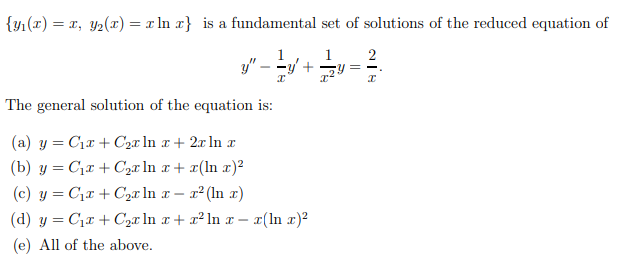{y () = r, yz(2) = r ln r} is a fundamental set of solutions of the reduced equation of y" - 12 Y+342 The general solution of the equation is: (a) y=CC + Cor In + 2.c in (b) y=C1+Cz.r In x + r(In c)? (c) y=C: +Car In 1 - (In r) (d) y=C2+ Cear In x + x? In z - (In )2 (e) All of the above. {yı(1) = 1, y2() = ??} is a fundamental...

• ### Consider the following 2nd order nonhomogeneous linear equation x 00 + 4x 0 + 5x =...Consider the following 2nd order nonhomogeneous linear equation x 00 + 4x 0 + 5x = cos 2t 1. Solve for the fundamental solutions of its associated homogeneous equation. 2. Find a particular solution of the nonhomogeneous equation. 3. Based on your answer to the previous two questions, write down the general solution of the nonhomogeneous equation. Problem II (15 points) Consider the following 2nd order nonhomogeneous linear equation x" + 40' + 5x = cos 2t 1. (6 points)...

• ### 5. Repeat the same questions in 4.) for the ODE Py"- tt+2)y+(t+2)y2t3, (t>0) (a) Find the general solution of the homogeneous ODE y"- 5y +6y 0. Particularly find yi and (b) Find the...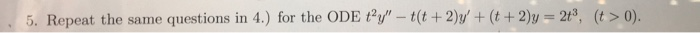5. Repeat the same questions in 4.) for the ODE Py"- tt+2)y+(t+2)y2t3, (t>0) (a) Find the general solution of the homogeneous ODE y"- 5y +6y 0. Particularly find yi and (b) Find the equivalent nonhomogeneous system of first order with the chan of variable y (c) Show that (nvand 2( re solutions of the homogeneous system of ODEs (d) Find the variation of parameters equations that have to be satisfic 1 for y(t) vi(t)u(t) + (e) Find the variation of...

• ### A) Assume that y1(c) t and y2)te are solutions of the differential equation t2y_ t(t + 2))" + t(t...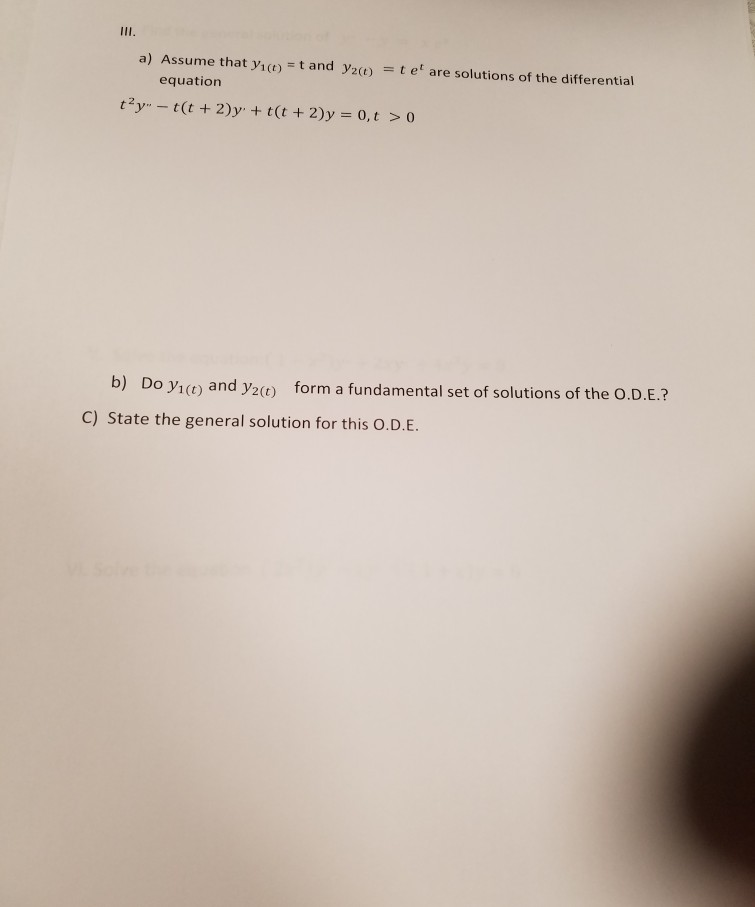a) Assume that y1(c) t and y2)te are solutions of the differential equation t2y_ t(t + 2))" + t(t + 2)y-0, t > 0 Do y1(t) and y2() form a fundamental set of solutions of the O.D.E.? C) State the general solution for this O.D.E. a) Assume that y1(c) t and y2)te are solutions of the differential equation t2y_ t(t + 2))" + t(t + 2)y-0, t > 0 Do y1(t) and y2() form a fundamental set of solutions of...

• ### Question 1 Let Y = 1 +2X + u where X = Zn4Z2, u = Z1-22, Z1 and Z2 are independent standard norma...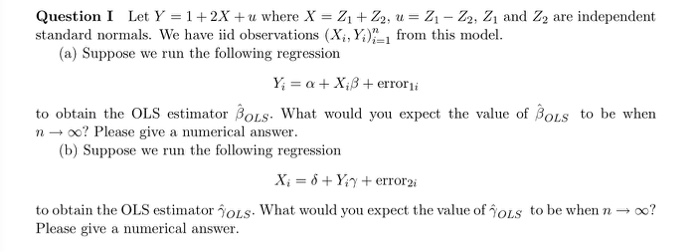Question 1 Let Y = 1 +2X + u where X = Zn4Z2, u = Z1-22, Z1 and Z2 are independent standard normals. We have iid observations (X,, Y1 from this model. (a) Suppose we run the following regression to obtain the OLS estimator β0Ls. What would you expect the value of β0LS to be when no Please give a numerical answer (b) Suppose we run the following regression to obtain the OLS estimator γ0Ls. What would you expect the...

• ### (1 point) The general solution of the homogeneous differential equation can be written as 2 where...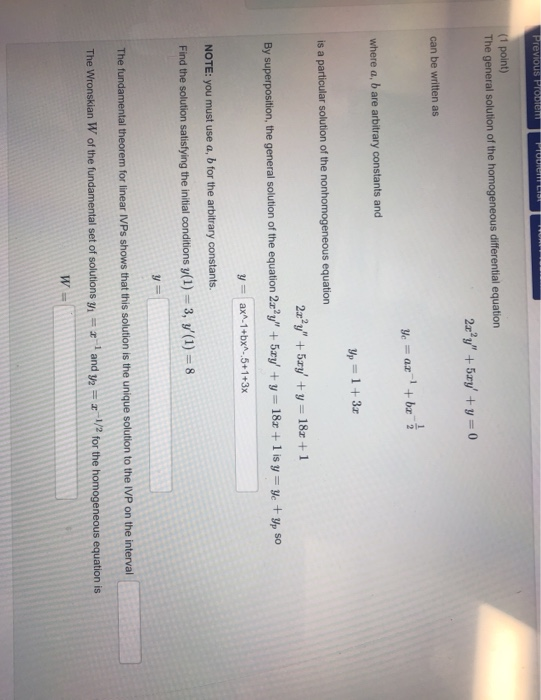(1 point) The general solution of the homogeneous differential equation can be written as 2 where a, b are arbitrary constants and is a particular solution of the nonhomogeneous equation By superposition, the general solution of the equation 2y 5ryy 18z+1 isyp so yax-1+bx-5+1+3x NOTE: you must use a, b for the arbitrary constants. Find the solution satisfying the initial conditions y(1) 3, y'(1) 8 The fundamental theorem for linear IVPs shows that this solution is the unique solution to...

• ### 3. Use reduction of order to find the fundamental set of solutions and write the general...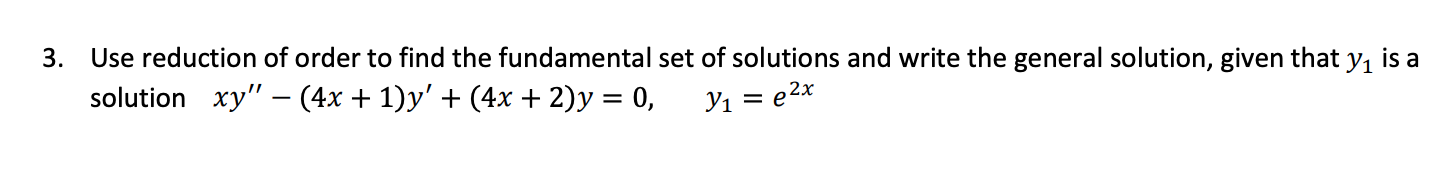3. Use reduction of order to find the fundamental set of solutions and write the general solution, given that y1 is a solution xy" – (4x + 1)y' + (4x + 2)y = 0, Y1 = e2x

• ### In space R^3, we define a scalar product by regulation 〈(x1, y1, z1), (x2, y2, z2)〉...

In space R^3, we define a scalar product by regulation 〈(x1, y1, z1), (x2, y2, z2)〉 = 2x1x2 + y1y2 + 2z1z2 + x1z2 + x2z1. (a)  Calculate the perpendicular projection of the point T (1, 1, 1) on the plane U in R3 with the equation x + 2y + 2z = 0 with respect to the given scalar product. (b)  Let φ: R^3 → R be a linear functional with φ (x, y, z) = x...

• ### A particular solution and a fundamental solution set are given for the nonhomgeneous equation below and...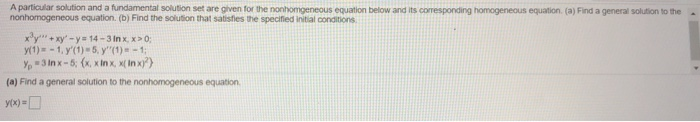A particular solution and a fundamental solution set are given for the nonhomgeneous equation below and its corresponding homogeneous equation (a) Find a general solution to the nonhomogeneous equation. (b) Find the solution that satisfies the specified initial conditions xy" + xy - y = 14 - 3 In x. x > 0; (1) - 1. y'(1) -5, y'(1) - - 1; y = 3 in x = 6; 8, 8 P 1, XÃ An xi} (a) Find a general...